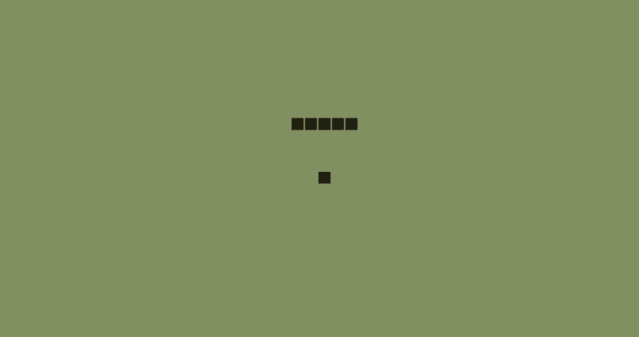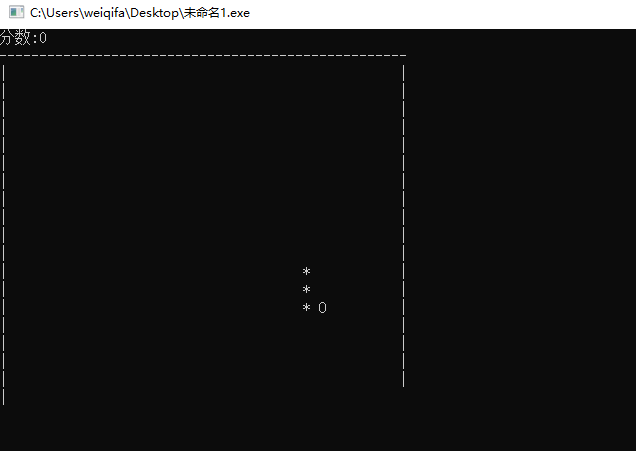# C语言写个贪吃蛇游戏menu();
setup();
draw();


typedef enum
{
STOP = 0,
LEFT,
RIGHT,
UP,
DOWN
}Direction;


/*判断贪吃蛇的长度*/
void logic()


/*把上一个位置记下*/
int lastX = tailX;
int lastY = tailY;
int last2X, last2Y;
/*重新获取当前的位置*/
tailX=x;
tailY=y;
int i=0;
/*遍历整条蛇的长度 把 0 的位置空出来，其余蛇的位置往后面的空间移动*/
for(i=1; i<ntail;i++)
{
last2X = tailX[i];
last2Y = tailY[i];
tailX[i]=lastX;
tailY[i]=lastY;
lastX = last2X;
lastY = last2Y;
}


lastX lastY 用来存上一次的蛇头的位置。后面的 for 循环，通过tail 蛇的长度，把蛇上个状态给保存到数组tailX tailY里面。


#include <stdio.h>
#include <stdlib.h>
#include <stdbool.h>
#include <Windows.h>
#include <time.h>
#include <conio.h> /*键盘输入获取*/

bool gameOver;
bool stop = false;
bool hit = false;
/*游戏的边框大小*/
const int width = 50;
const int height = 20;
/*蛇的坐标，食物的坐标还有分数*/
int x,y,fruitX,fruitY,score;
/*蛇每个点的坐标*/
int tailX,tailY;
/*蛇的默认长度*/
int ntail=3;

typedef enum
{
STOP = 0,
LEFT,
RIGHT,
UP,
DOWN
}Direction;

Direction Dir;
/*开始菜单*/
{
int a;
printf("------------------------------------------------------------------\n");
printf("|                              贪吃蛇游戏                        |\n");
printf("|                              1) 新游戏                         |\n");
printf("|                              2) 开始边界                       |\n");
printf("|                              3) 退出游戏                       |\n");
printf("------------------------------------------------------------------\n");
printf("---->请输入你的选择:");
scanf("%d", &a);
}

/*初始化状态*/
void setup()
{
gameOver = false;
/*根据当前时间设置“随机数种子”*/
srand(time(NULL));
Dir = STOP;

/*贪吃蛇的位置,固定在中间*/
x= width/2;
y= height/2;
/*食物的位置，位置是随机的*/
fruitX = rand()%width;
fruitY = rand()%height;
score = 0;
}
/*绘制界面*/
void draw()
{
if(stop == true)
{
return;
}
system("cls");/*清除屏幕*/
printf("分数:%d",score);
printf("\n");

/*第一行*/
int i;
for(i= 0 ;i<width+1;i++)
{
printf("-");
}
printf("\n");

/*画中间的画面*/
int p;
for(p= 0 ;p<height;p++)/*高度*/
{
int q;
for(q= 0 ;q<width;q++)/*宽度*/
{
/*第一行最后已给字符*/
if(q==0 || q==width-1)
{
printf("|");
}

if(p == fruitY && q == fruitX)/*食物的随机坐标*/
{
printf("O");
}
else
{
int k=0;
bool print = false;
/*贪吃蛇的长度 默认长度是 3*/
for(k=0;k<ntail;k++)
{
if(tailX[k]==q && tailY[k]==p)
{
printf("*");
print = true;
}
}
/*如果这个位置打印了 * 就不要打印空格了*/
if(!print)
{
printf(" ");
}
}
}
printf("\n");
}

/*最后一行*/
int j;
for(j= 0 ;j<width+1;j++)
{
printf("-");
}

}
/*按键输入控制*/
void input()
{
if(_kbhit())
{
/*获取键盘的输入字符*/
switch(_getch())
{
case '4':
case 75:/*左键*/
Dir = LEFT;
hit= true;
break;
case '8':
case 72:/*上键*/
Dir = UP;
hit= true;
break;
case '6':
case 77:/*右键*/
Dir = RIGHT;
hit= true;
break;
case '2':
case 80:/*向下键盘键 */
Dir = DOWN;
hit= true;
break;
case 'x':
case 27:/*ESE*/
gameOver = true;
break;
case 32:/*空格 暂停键*/
stop = !stop;
break;
}
}
else if(!hit && stop == false)/*如果没有改变方向*/
{
x++;
}
}
/*判断贪吃蛇的长度*/
void logic()
{
if(stop == true)
{
return;
}

/*把上一个位置记下*/
int lastX = tailX;
int lastY = tailY;
int last2X, last2Y;
/*重新获取当前的位置*/
tailX=x;
tailY=y;
int i=0;
/*遍历整条蛇的长度 把 0 的位置空出来，其余蛇的位置往后面的空间移动*/
for(i=1; i<ntail;i++)
{
last2X = tailX[i];
last2Y = tailY[i];
tailX[i]=lastX;
tailY[i]=lastY;
lastX = last2X;
lastY = last2Y;
}
/*根据方向来改变x y 的值*/
switch(Dir)
{
case UP:
y--;
break;
case DOWN:
y++;
break;
case LEFT:
x--;
break;
case RIGHT:
x++;
break;
}
if(x<0 || width<x || y<0 || height<y)
{
gameOver = true;
/*清除屏幕*/
system("cls");
printf("------------------------------------------------------------------\n");
printf("|                                                                |\n");
printf("|                                                                |\n");
printf("|                             游戏结束                           |\n");
printf("|                                                                |\n");
printf("|                                                                |\n");
printf("------------------------------------------------------------------\n");
}
if(x==fruitX && y==fruitY)
{
/*吃了一个食物，蛇的长度增加1*/
ntail++;
score+=10;
/*更新下一个食物的位置*/
fruitX = rand()%width;
fruitY = rand()%height;
}
}
int main()
{
#if 0
while(1)
{
printf("%d\n",_getch());
}
#endif
setup();
draw();
/*循环画贪吃蛇的界面*/
while(!gameOver)
{
draw();
input();
logic();
Sleep(70);
}

return 0;
}©️2019 CSDN 皮肤主题: 数字50 设计师: CSDN官方博客